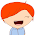## Sunday, January 25, 2015

### Odds, odds ratio, language and intuition

I was reading a statistics book the other day. I am at the beginning. In this section I read '(we) report results as odds ratios, which is more intuitive'. I must have read sentences stating this any number of times. But I don't agree.
It may be my background. My mother tongue is Dutch, which does not have a word for odds. Hence odds is a word which I only learned when I got into statistics. It is an abstract mathematical construct. The odds ratio then, is a ratio between two abstract numbers and initially was just a number. Since I never did much in odds and odds ratio, they never gained meaning either.
Now I am just wondering, what proportion of people reading this kind of books does not have an intuition for odds?

1.(I think my previous comment got swallowed).

I don't think people who are neither statisticians nor gamblers have a good sense for odds (or log-odds), regardless of whether they have a word for "odds" in their language ... I would love to see more answers here about good resources for teaching these ideas/intuitions, e.g. along the lines of http://www.ats.ucla.edu/stat/mult_pkg/faq/general/odds_ratio.htm , http://www.theanalysisfactor.com/why-use-odds-ratios/ http://www.ncbi.nlm.nih.gov/pubmed/22194149 (paywalled) ...

2.I think it's difficult to intuit odds ratios, and that's one reason it's difficult to put meaningful priors on regression coefficients in logistic regression, and that's why it's useful to actually look at an MCMC sample from the prior logistic regression, to see that it's reasonable.

3.In previous comment I meant: look at an MCMC sample of the prior on mu, mu=logistic(b0+b1*x1+...). Chapter 21 of DBDA2E has examples.

4.I agree. It can be difficulty to intuitively understand odds ratios--particularly for students in the social sciences. This is a nice little article I've found helpful when teaching probabilities, odds, and odds ratios: http://www.csrpsp.com/bg/upload/40041bae-5ecd-4734-b5fd-1fec3592945d.pdf

5.Another article I've found useful is this one:
http://www.bmj.com/content/316/7136/989
Figure 2 shows the relationship between risk and odds in different situations.

6.Not sure if my prev comment went through or the auth change killed it.

I really find odds tough to use day to day. I use binomial logistic regressions quite often so the logits and subsequent odds don't have a huge amount of meaning to me. I need to bring them into probability terms so I have a bunch of helper functions in my package optiRum to take care of these for me.

7.The funny thing is that if you transform your raw probabilities into log-odd-rations you often see linear relationships in your data. Somehow reality likes log-odd-ratios. An intuitive explanation would be nice!

8.I also think that odds are less intuitive as mathematics instruction has moved further away from fractions and finding remainders and using decimal approximations in calculations. People are just more comfortable with decimal expansions (not necessarily competent, but comfortable) since the use of calculators and computers. Unless you gamble.